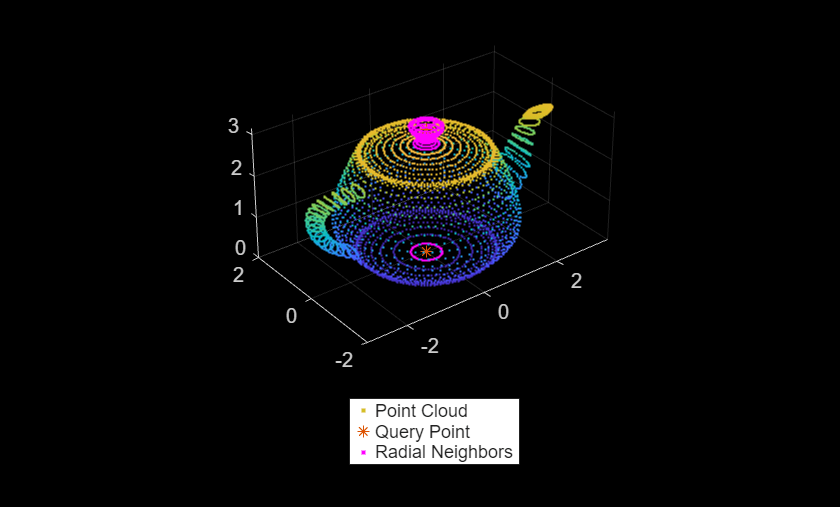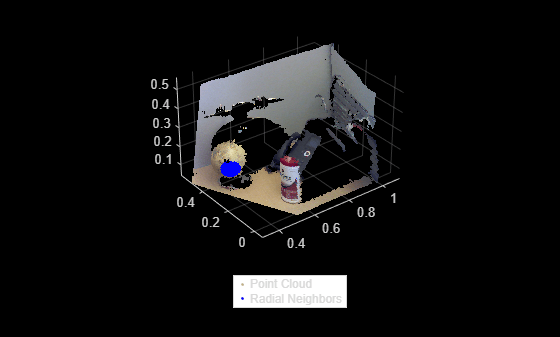Find neighbors within a radius of a point in the point cloud

## Syntax

``[indices,dists] = findNeighborsInRadius(ptCloud,point,radius)``
``[indices,dists] = findNeighborsInRadius(ptCloud,point,radius,camMatrix)``
``[indices,dists] = findNeighborsInRadius(___,Name,Value)``

## Description

example

````[indices,dists] = findNeighborsInRadius(ptCloud,point,radius)` returns the `indices` of neighbors within a radius of a query point in the input point cloud. `ptCloud` can be an unorganized or organized point cloud. The neighbors within a radius of the query point are computed by using the Kd-tree based search algorithm.```

example

````[indices,dists] = findNeighborsInRadius(ptCloud,point,radius,camMatrix)` returns the neighbors within a radius of a query point in the input point cloud. The input point cloud is an organized point cloud generated by a depth camera. The neighbors within a radius of the query point are determined using fast approximate neighbor search algorithm. The function uses the camera projection matrix `camMatrix` to know the relationship between adjacent points and hence, speeds up the search. However, the results have lower accuracy as compared to the Kd-tree based approach. NoteThis syntax only supports organized point cloud data produced by RGB-D sensors.You can use `estimateCameraMatrix` to estimate camera projection matrix for the given point cloud data. ```
````[indices,dists] = findNeighborsInRadius(___,Name,Value)` specifies options using one or more name-value pair arguments in addition to the input arguments in the preceding syntaxes.```

## Examples

collapse all

Load a set of 3-D coordinate points into the workspace.

`load('xyzPoints.mat');`

Create a point cloud object.

`ptCloud = pointCloud(xyzPoints);`

Specify a query point and the radius within which the neighbors are to be identified.

```point = [0,0,3]; radius = 0.5;```

Get the indices and the distances of points that lie within the specified radius.

`[indices,dists] = findNeighborsInRadius(ptCloud,point,radius);`

Get the point cloud data of radial neighbors.

`ptCloudB = select(ptCloud,indices);`

Display the point cloud. Plot the query point and the corresponding radial neighbors.

```figure pcshow(ptCloud) hold on plot3(point(1),point(2),point(3),'*') pcshow(ptCloudB.Location,'r') legend('Point Cloud','Query Point','Radial Neighbors','Location','southoutside','Color',[1 1 1]) hold off```Find radial neighbors of a query point in the organized point cloud data by using the camera projection matrix. Compute the camera projection matrix from sampled point cloud data points and their corresponding image point coordinates.

Load an organized point cloud data into the workspace. The point cloud is generated by using the Kinect depth sensor.

```ld = load('object3d.mat'); ptCloud = ld.ptCloud;```

Specify the step size for sampling the point cloud data.

`stepSize = 100;`

Sample the input point cloud and store the sampled 3-D point coordinates as a point cloud object.

```indices = 1:stepSize:ptCloud.Count; tempPtCloud = select(ptCloud,indices);```

Remove invalid points from the sampled point cloud.

`[tempPtCloud,validIndices] = removeInvalidPoints(tempPtCloud);`

Define the 3-D world point coordinates of input point cloud.

`worldPoints = tempPtCloud.Location;`

Find the 2-D image coordinates corresponding to the 3-D point coordinates of input point cloud.

```[Y,X] = ind2sub([size(ptCloud.Location,1),size(ptCloud.Location,2)],indices); imagePoints = [X(validIndices)' Y(validIndices)'];```

Estimate camera projection matrix from the image and the world point coordinates.

`camMatrix = estimateCameraMatrix(imagePoints,worldPoints);`

Specify a query point and the radius within which the neighbors are to be identified.

```point = [0.4 0.3 0.2]; radius = 0.05;```

Get the indices and the distances of radial neighbors. Use the point cloud method `select` to get the point cloud data of neighboring points.

```[indices,dists] = findNeighborsInRadius(ptCloud,point,radius,camMatrix); ptCloudB = select(ptCloud,indices);```

Display the point cloud and the radial neighbors found around a query point.

```figure pcshow(ptCloud); hold on; pcshow(ptCloudB.Location, 'b'); legend('Point Cloud','Radial Neighbors','Location','southoutside','Color',[1 1 1] ) hold off;```## Input Arguments

collapse all

Point cloud, specified as a `pointCloud` object.

Query point, specified as a three-element vector of form `[x,y,z]`.

Search radius, specified as a scalar. The function finds the neighbors within the specified `radius` around a query point in the input point cloud.

Camera projection matrix, specified as a 4-by-3 matrix that maps 3-D world points to 2-D image points. You can find `camMatrix` by using the `estimateCameraMatrix` function.

### Name-Value Arguments

Specify optional comma-separated pairs of `Name,Value` arguments. `Name` is the argument name and `Value` is the corresponding value. `Name` must appear inside quotes. You can specify several name and value pair arguments in any order as `Name1,Value1,...,NameN,ValueN`.

Example: `findNeighborsInRadius(ptCloud,point,radius,'Sort',true)`

Sort indices, specified as a comma-separated pair of `'Sort'` and a logical scalar. When you set `Sort` to `true`, the returned indices are sorted in the ascending order based on the distance from a query point. To turn off sorting, set `Sort` to `false`.

Number of leaf nodes, specified as a comma-separated pair consisting of `'MaxLeafChecks'` and an integer. When you set this value to `Inf`, the entire tree is searched. When the entire tree is searched, it produces exact search results. Increasing the number of leaf nodes to check increases accuracy, but reduces efficiency.

## Output Arguments

collapse all

Indices of stored points, returned as a column vector. The vector contains the linear indices of the radial neighbors stored in the point cloud.

Distances to query point, returned as a column vector. The vector contains the Euclidean distances between the query point and its radial neighbors.

 Muja, M. and David G. Lowe. "Fast Approximate Nearest Neighbors with Automatic Algorithm Configuration". In VISAPP International Conference on Computer Vision Theory and Applications. 2009. pp. 331–340.